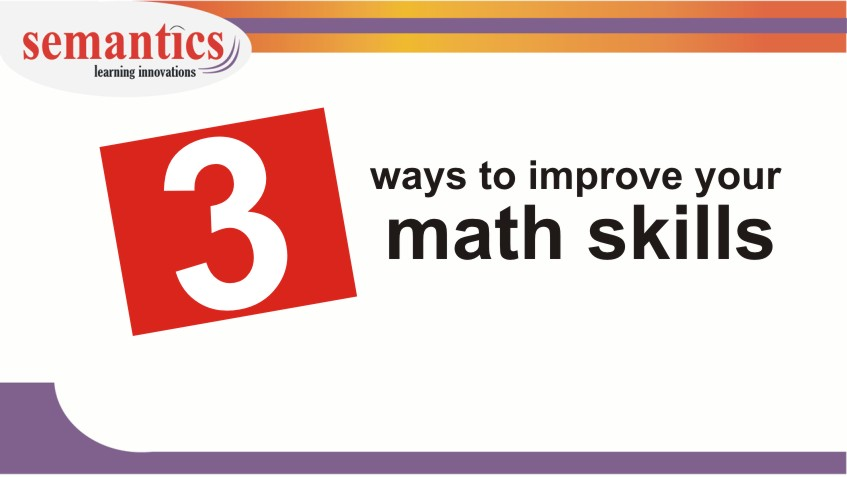# 3 ways to improve your math skillsMath is tough for some of us.

The reason – The way math was taught to us in school or college

Can it be mastered now?

Yes, you can….

The exam tests your mathematical reasoning.

• reason with numbers and symbols.
• apply principles/formula and solve questions
• evaluate cases or arguments
• strategize for a solution
• identify patterns and use logic
• check data efficacy and more.

So if you are taught skills ( Skills are different from math concepts), you will be fine.

Ping us if you would like to know more about the

### Click the form here.

So how we do work on our math skills. Here are 3 ways, which can help you hone your general math skills

## 1.Make math part of your daily life... practice it without even thinking.

For example, if a dress that’s regularly \$50 is on sale for 30% off, what is the sale price?

Do simple calculation.

Or if you have to travel 20 miles. You are going at a speed of 20 miles per hour. How long will you take?

## 2.Use mental math everyday.

20+30+45+60 = ??? don’t use calculator.

what is 15 squared or what is 20 squared…

Everyday spend 5 – 10 minutes working on basic computation

## 3.Practice diagramming.

Always picture a math problem.

Make few circles/ draw connections/ some stick figures….

Here are sample images for math problems

If you have any queries regarding math preparation, get in touch with us

Happy learning..

Call Now Button